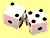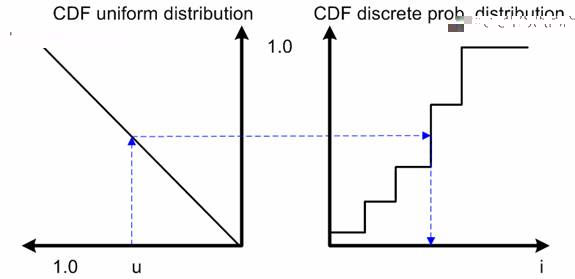## Nuts and Bolts of Monte Carlo Simulation

Monte Carlo Simulation concerns with generation of random numbers from some given distribution. Most computer programming languages or spreadsheets have built in function to generate uniform distribution random number that can be easily used. For example, use = RAND () function in MS excel or RND in Visual Basic to generate random number between zero and one.

The principle behind Monte Carlo simulation is to generate other type of distribution based on uniform distribution random number. It requires a transformation from uniform distribution into other distribution.For example, diagram above show how uniform random distribution is transformed by the discrete probability distribution to represent the later distribution.

In the next few pages, you will learn how to do this transformation. Before that, you may want to know how your computer generate random number according to uniform random distribution

Preferable reference for this tutorial is

Teknomo, Kardi. Monte Carlo Simulation Tutorial. http://people.revoledu.com/kardi/ tutorial/Simulation